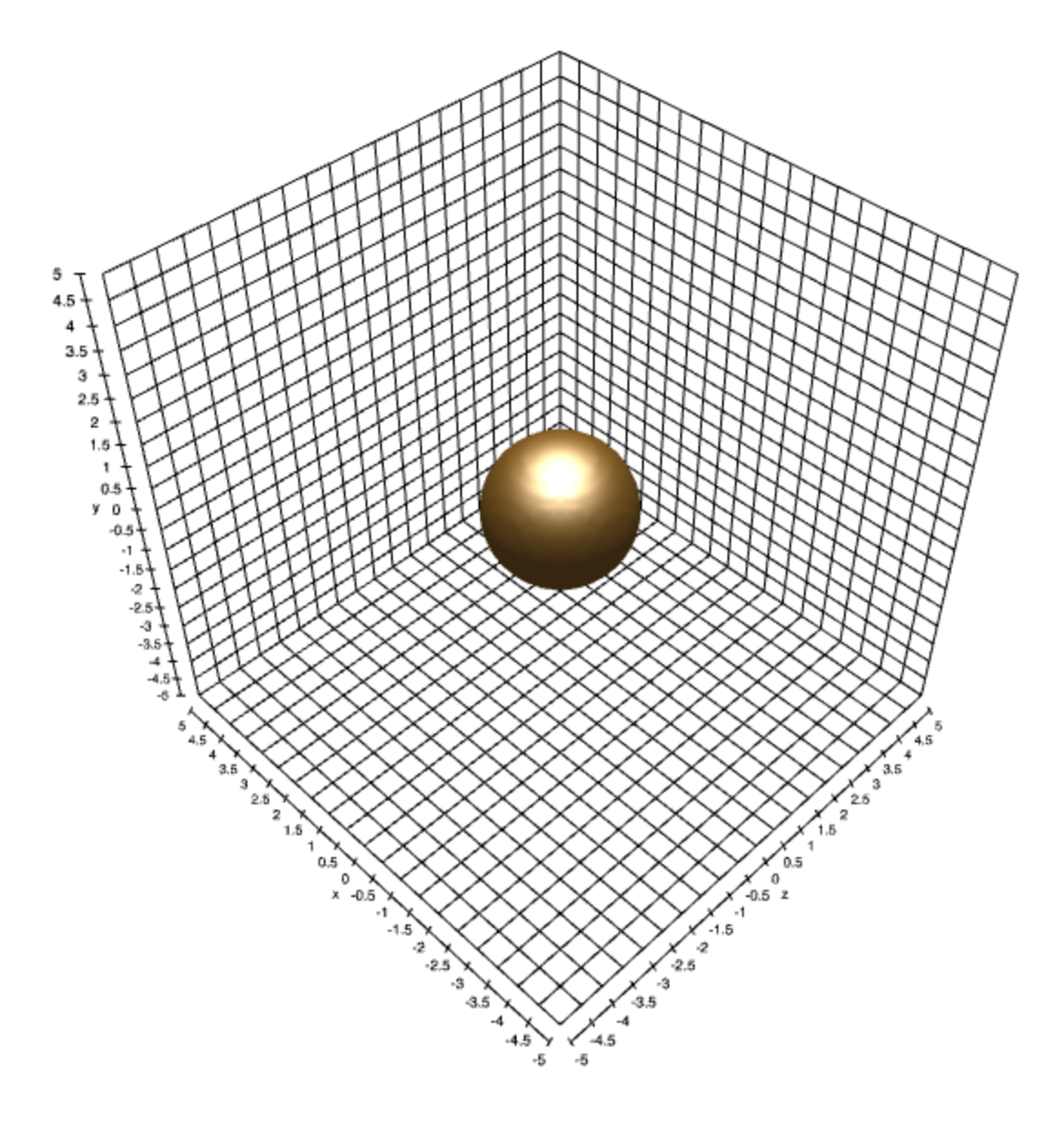Neon Power Mains

npm

gl-axes

6.0.2 • Public • Published

gl-axes

Draws axes for 3D scenes:Example

Here is a simple example showing how to use gl-axes to visualize the extents of an isosurface:

You can play with this demo yourself on requirebin.

Install

npm install gl-axes

API

Constructor

var axes = require("gl-axes")(gl[, params])

Creates an axes object.

• gl is a WebGL context
• params is an object with the same behavior as axes.update

Returns A new glAxes object for drawing the

Methods

axes.draw(camera)

Draws the axes object with the given camera parameters. The camera object can have the following properties:

• model - Is the model matrix for the axes object (default identity)
• view - Is the view matrix for the axes (default identity)
• projection - Is the projection matrix for the axes (default identity)

All camera matrices are in 4x4 homogeneous coordinates and encoded as length 16 arrays as done by gl-matrix.

axes.update(params)

Updates the parameters of the axes object using the properties in params. These are grouped into the following categories:

Dimensions and tick lines

• bounds the bounding box for the axes object, represented as a pair of 3D arrays encoding the lower and upper bounds for each component. Default is [[-10,-10,-10],[10,10,10]]
• tickSpacing either a number or 3d array representing the spacing between the tick lines for each axis. Default is 0.5
• ticks Alternatively, you can specify custom tick labels for each axis by passing in an array of 3 arrays of tick markings. Each tick marking array is an array of objects with the properties x and text which denote the position on the tick axis and the text of the tick label respectively.

Tick text

• tickEnable a boolean value (or array of boolean values) which selects whether the tick text is drawn. Default true
• tickFont a string (or array of strings) encoding the font style for the tick text. Default 'sans-serif'
• tickSize the size of the font text in pixel coordinates. Default is computed from tick spacing.
• tickAngle a number (or array of numbers) encoding the angle of the tick text with the vertical axis in radians. Default 0
• tickColor a color (or array of colors) indicating the color of the text for each tick axis. Default [0,0,0]
• tickPad a number (or array of numbers) encoding the world coordinate offset of the tick labels from tick marks. Default 1

Labels

• labelEnable a boolean value or array of boolean
• labelText a 3D array encoding the labels for each of the 3 axes. Default is ['x', 'y', 'z']
• labelFont a string (or array of strings) representing the font for each axis. Default 'sans-serif'
• labelSize the size of the label text in pixel coordinates. Default is computed from tick spacing
• labelAngle a number (or array of numbers) encoding the angle of the label text with the vertical axis in radians. Default 0
• labelColor a color array (or array of color arrays) encoding the color of the label for each axis. Default [0,0,0]
• labelPad a number (or array of numbers) encoding the world coordinate offset of the labels from the data axes. Default 1.5

Axis lines

• lineEnable a boolean (or array of booleans) determining which of the 3 axes tick lines to show. Default is true
• lineWidth a number (or array of numbers) determining the width of the axis lines in pixel coordinates. Default is 1
• lineMirror a boolean (or array of booleans) describing which axis lines to mirror. Default is false
• lineColor a color array (or array of color arrays) encoding the color of each axis line. Default is [0,0,0]

Axis line ticks

• lineTickEnable a boolean (or array of booleans) which determines whether or not to draw the line ticks. Default is false
• lineTickMirror a boolean (or array of booleans) which determines whether the line ticks will be mirrored (similar behavior to lineMirror). Default is false
• lineTickLength a number (or array of numbers) giving the length of each axis tick in data coordinates. If this number is positive, the ticks point outward. If negative, the ticks point inward. If 0, the tick marks are not drawn. Default 0
• lineTickWidth a number (or array of numbers) giving the width of the tick lines pixel coordinates. Default 1
• lineTickColor a color (or array of colors) giving the color of the line ticks. Default [0,0,0]

Grid lines

• gridEnable a boolean (or array of booleans) determining which grid lines to draw. Default is true
• gridWidth a number (or array of numbers) giving the width of the grid lines in pixel units. Default is 1
• gridColor a color array (or array of color arrays) giving the color of the grid lines for each axis. Default is [0,0,0]

Zero line

• zeroEnable a boolean (or array of booleans) which describes which zero lines to draw. Default is true
• zeroLineColor a color array (or array of color arrays) giving the color of the zero line. Default is [0,0,0]
• zeroLineWidth a number (or array of numbers) giving the width of the zero line. Default is 2

Background

• backgroundEnable a boolean (or array of booleans) describing which background plane to draw. Default is false
• backgroundColor the color of each background plane. Default is [0.8,0.8,0.8,0.5]

axes.dispose()

Releases all resources associated with this axes object.

Credits

(c) 2014 Mikola Lysenko. MIT License

Keywords

Install

npm i gl-axes